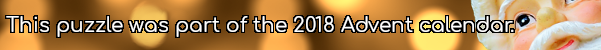mscroggs.co.uk
mscroggs.co.uksubscribe

## 22 DecemberIn base 2, 1/24 is 0.0000101010101010101010101010...
In base 3, 1/24 is 0.0010101010101010101010101010...
In base 4, 1/24 is 0.0022222222222222222222222222...
In base 5, 1/24 is 0.0101010101010101010101010101...
In base 6, 1/24 is 0.013.
Therefore base 6 is the lowest base in which 1/24 has a finite number of digits.
Today's number is the smallest base in which 1/10890 has a finite number of digits.
Note: 1/24 always represents 1 divided by twenty-four (ie the 24 is written in decimal).

## Archive

Show me a random puzzle
▼ show ▼